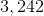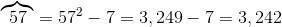# ISEE Lower Level Quantitative : How to multiply

## Example Questions

← Previous 1 3 4 5 6 7 8 9 24 25

### Example Question #1 : How To Multiply

The grocery store has 34 boxes of cookies on the shelf.  Each box contains 20 cookies.  Which expression shows how many total cookies are on the shelf?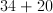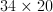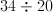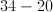Explanation:

There are 20 cookies in each box, and there are 34 boxes.  We need to multiply the number of cookies in each box by the number of boxes.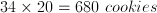### Example Question #2 : How To Multiply

If Lisa hasboxes of pens, and each box contains 12 pens, which expression would tell us how many pens that Lisa has?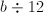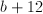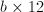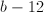Explanation:

Every box of pens represents 12 total pens.  So if we have 3 boxes, we have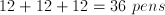which is the same as saying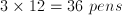.

Here, since we only know that Lisa hasboxes of pens, so we would multiply that by the 12 pens in each box.

### Example Question #3 : How To Multiply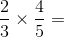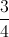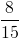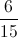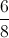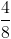Explanation:To multiply fractions, we multiply the numerators together first.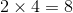Then, we multiply the denominators together..

Then we divide the first answer (8) by the second answer (15) to get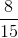.

### Example Question #1 : How To Multiply

Find the product.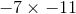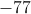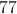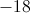Explanation:

When multiplying two negative numbers, the product will be positive. The correct answer is 77.

### Example Question #5 : How To Multiply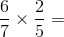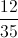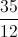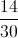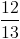Explanation:

To get the answer, you multiply the numerators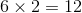and then the denominators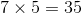to get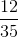### Example Question #3 : How To Multiply

Sally Student has 12 boxes of pencils, with 10 pencils in each box. Which expression shows how many pencils she has total?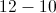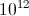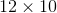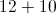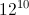Explanation:

In order to calculate how many pencils there are total, one must multiply the number of pencils in each box by the number of boxes.

### Example Question #4 : How To Multiply

Maggie is making cookies for her friends. The recipe calls forcup of sugar, and three times as much flour.

How many total cups of sugar and flour will she need if she quadruples the recipe?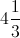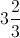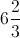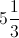Explanation: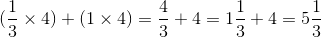### Example Question #8 : How To Multiply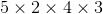is equal to the product ofand what number?Explanation: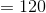Therefore, one needs to find the number that can be multiplied byto yield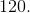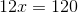The best way to do this is to divideby.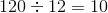### Example Question #5 : How To Multiply

Maggie is making cookies for her friends. The recipe calls forcup of sugar, and three times as much flour.

How many cups of flour does Maggie need for her recipe?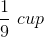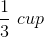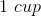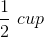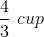Explanation: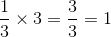### Example Question #6 : How To Multiply

Define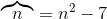.

Evaluate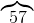.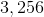The expression is undefined.